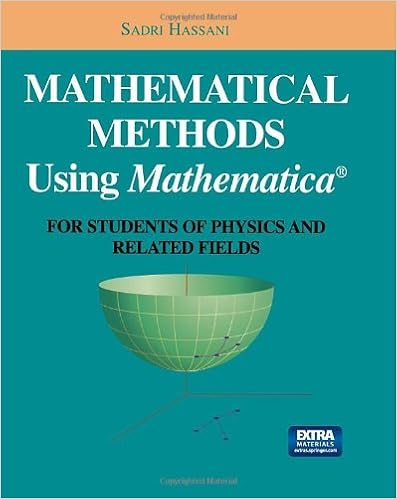Tuesday, August 4, 2015

Mathematical Methods Using Mathematica®: For Students of Physics and Related Fields (Undergraduate Texts in Contemporary Physics) by Sadri HassaniIntended as a companion for textbooks in mathematical methods for science and engineering, this book presents a large number of numerical topics and exercises together with discussions of methods for solving such problems using Mathematica(R). Although it is primarily designed for use with the author's "Mathematical Methods: For Students of Physics and Related Fields," the discussions in the book sufficiently self-contained that the book can be used as a supplement to any of the standard textbooks in mathematical methods for undergraduate students of physical sciences or engineering.